• 数据结构迷宫问题c++
千次阅读
2017-05-27 22:27:52

出现实现图：.h文件实现堆栈简易操作（此处没有将声明与实现分离，应注意！）

#pragma warning (disable : 4715)
#ifndef  STACK_H
#define STACK_H
struct Position//结构体位置，表示迷宫每一格的行号和 列号
{
int row;//行号
int col;//列号
};
class Stack
{
public:
Stack(int MaxSize = 10);
~Stack() { delete[] Element; };
Position Top() const;//返回栈顶元素
Stack& Delete(Position &x);//删除元素
private:
int top;//栈顶，入栈和出栈的地方。
int Maxtop;//栈顶元素位置。
Position *Element;
};
Stack::Stack(int MaxSize)
{
Maxtop = MaxSize - 1;
Element = new Position[MaxSize];
top = -1;
}
Position Stack::Top() const
{
if (IsEmpty())
{
}
else
return Element[top];
}
{
if (IsFull())
{
}
else
{
++ top;
Element[top].row = x.row;
Element[top].col = x.col;
}
return *this;
}
Stack& Stack::Delete(Position &x)
{
if (IsEmpty())
{
}
else
{

x.row = Element[top].row;
x.col = Element[top].col;
--top;
}
return *this;
}
#endif 

源文件：

#include<iostream>
#include"STack.h"
using namespace std;
int main()
{
cout << "老鼠迷宫！" << endl;
//构建迷宫。maze表示入口， maze表示出口。
char **maze = new char *;
for (int i = 0; i < 12; ++i)
maze[i] = new char;

//给迷宫加“墙”。
for (int i = 0; i < 12; ++i)
{
maze[i] = '+';
maze[i] = '+';
maze[i] = '+';
maze[i] = '+';
}
//构建迷宫，用符号+表示墙壁，空格表示通路，以使迷宫尽可能好看！
for (int a = 1; a < 11; ++a)
for (int b = 1; b < 11; ++b)
maze[a][b] = ' ';
for (int i = 2; i < 7; ++i)
maze[i] = '+';
maze = maze = maze = maze = maze = maze = maze = maze = maze = '+';
maze = maze = maze = maze = maze = maze = maze = maze = maze = maze = '+';
maze = maze = maze = maze = maze = maze = maze = maze = maze = '+';
maze = maze = maze = maze = maze = maze = '+';
//输出迷宫 布局。
cout << "迷宫如下所示：" << endl;
for (int a = 0; a < 12; ++a)
{
for (int b = 0; b < 12; ++b)
cout << maze[a][b] << " ";
cout << endl;
}
//构建迷宫完毕，开始寻找路径。
Stack* Path = new Stack(10*10 - 1);//栈用来储存路径以及遇到障碍物时方标返回上一步。

Position offset;//设置模拟移动方法。
offset.row = 0; offset.col = 1;//右移
offset.row = 1; offset.col = 0;//下移
offset.row = 0; offset.col = -1;//左移
offset.row = -1; offset.col = 0;//上移

Position here;//代表当前位置。
here.col = 1;
here.row = 1;
maze = '#';//入口堵住，防止返回入口。
int option = 0;//上、下、左、右四种走法。记录走的次数，一共四次，上下左右分布探索。
int Lastoption = 3;
//模拟移动，开始寻找出口。
while (here.row != 10 || here.col != 10)
{
int x, y;
while (option <= Lastoption)//最多循环4次，尝试每种走法。
{
x = here.row + offset[option].row;
y = here.col + offset[option].col;
if (maze[x][y] == ' ')//只要寻找得到通路，就退出循环。
break;
option++;
}
if (option <= Lastoption)
{//移动一次，是通路，此处位置入栈，移动数option归0。
here.row = x;
here.col = y;

maze[x][y] = '*';//将该处修改为墙壁，防止返回，做重复工作。
option = 0;
}
else//不是通路，会退一步，路径记录栈栈顶元素出栈。
{
if (Path->IsEmpty())//出现空栈，表明该迷宫没有出口！
cout << "这个迷宫没有出口！" << endl;
Position next;
next.col = next.row = 0;
Path->Delete(next);//新的位置，表示here的前一步，用来回退。

if (next.row == here.row)
option = 2 + next.col - here.col;//通过计算得出回退后应该执行哪一种走法！
else
option = 3 + next.row - here.row;
here = next;
}
}
cout << "老鼠找到了迷宫的出口！！" << endl;//以图形的样式输出寻找得到的路径。
for (int i = 0; i < 12; ++i)
{
for (int a = 0; a < 12; ++a)
cout << maze[i][a] << " ";
cout << endl;
}
system("pause");
}算法
更多相关内容
• 数据结构课程设计之C++编写的迷宫问题路径求解程序，使用的是栈方法，即将路径上每一步存在栈中，迷宫文件格式见程序提示，压缩包内已经给出了三个测试用的迷宫地图可用来测试，支持分步显示查找路径过程功能，当给...
• 数据结构迷宫问题C++源代码实现，对刚开始学习数据结构的人有帮助
• 这个文档详细的介绍了利用栈和队列解决迷宫问题的步骤，对与初学者学习数据结构能很好的进行辅导
• C++ 数据结构迷宫实验

给定一迷宫，1代表障碍物，0代表可通节点，求一条从迷宫入口到迷宫出库的路径。
本算法设定迷宫大小为Y_MAX*X_MAX,入口为，出口为[Y_MAX-2][X_MAX-2]

在每个节点时依次按照下、右、上、左的顺序寻找下个节点能否通过。
具体实现见代码，下为实际运行效果图。

当迷宫存在通路时: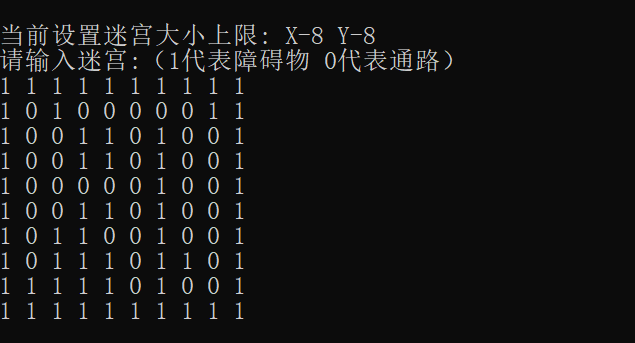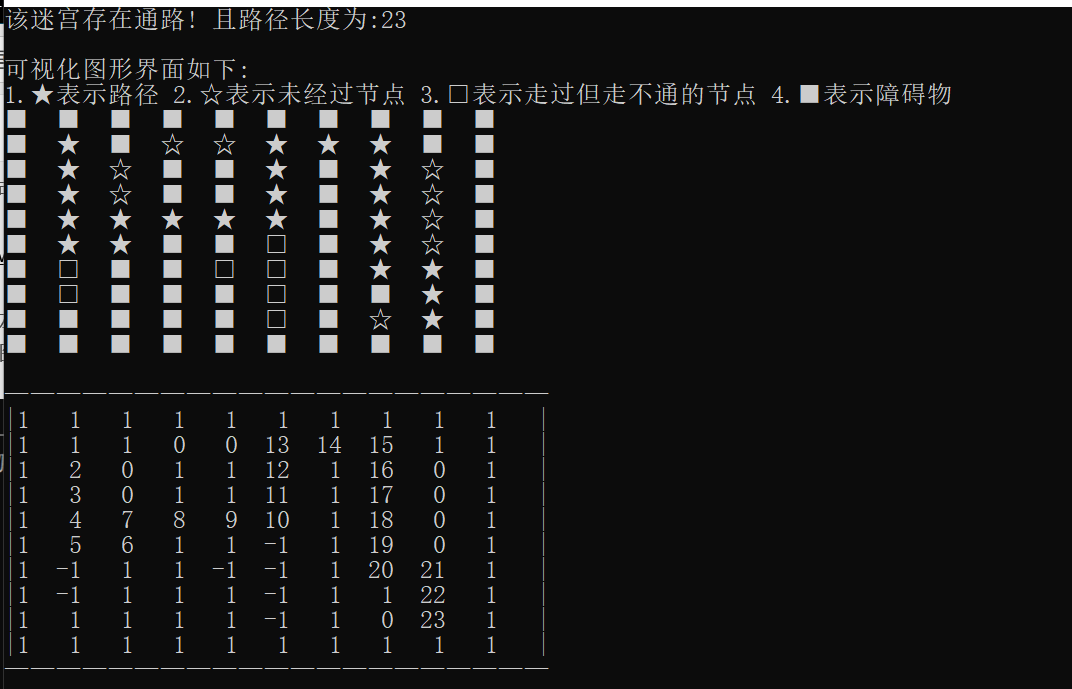当迷宫不存在通路时：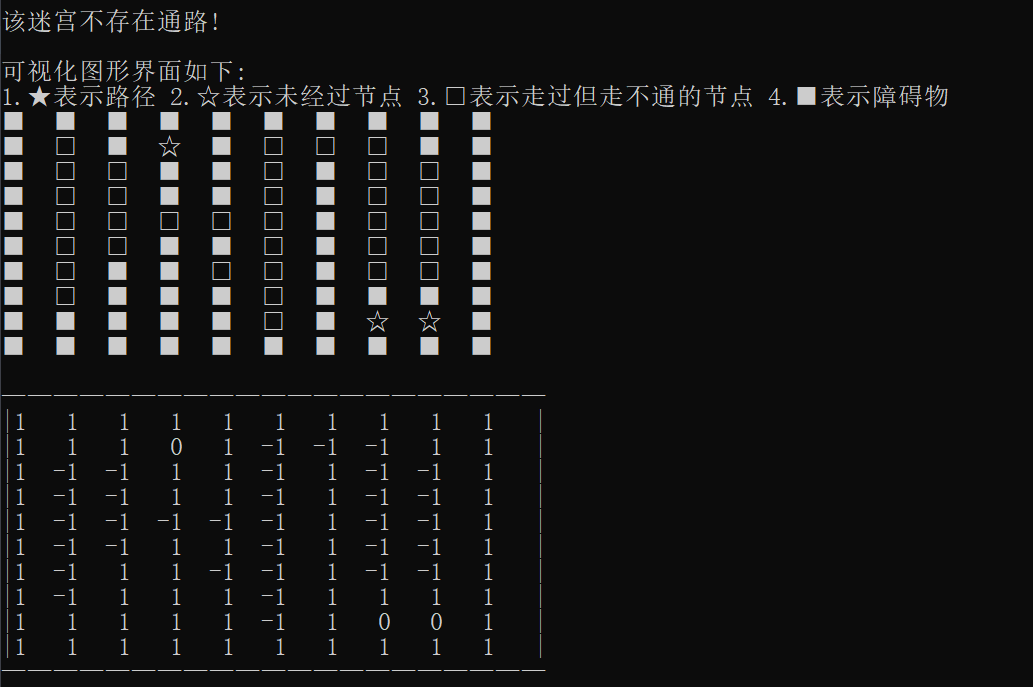//转载请标明出处 Postlude
#include <iostream>
#include <stack>
#include <iomanip>

using namespace std;

#define X_MAX 10
#define Y_MAX 10

int map[Y_MAX][X_MAX];
int flag = 0;

void get_map()
{
for (int i = 0; i < Y_MAX; i++)
for (int j = 0; j < X_MAX; j++)
cin >> map[i][j];
}

void print_map()
{
if (flag)
cout << "\n\n该迷宫存在通路! 且路径长度为:" << map[Y_MAX - 2][X_MAX - 2] << endl;
else
cout << "\n\n该迷宫不存在通路!\n";
cout << "\n可视化图形界面如下:\n1.★表示路径 2.☆表示未经过节点 3.□表示走过但走不通的节点 4.■表示障碍物\n";
for (int i = 0; i < Y_MAX; i++)
{
for (int j = 0; j < X_MAX; j++)
{
if (i == 1 && j == 1 && !flag)
{
cout << "□  ";
continue;
}
if (i == 1 && j == 1 && flag)
{
cout << "★  ";
continue;
}

if (map[i][j] == -1)
cout << "□  ";
else if (map[i][j] == 1)
cout << "■  ";
else if (map[i][j] == 0)
cout << "☆  ";
else
cout << "★  ";
}
cout << endl;
}
cout << "\n—————————————————————"<<endl;
for (int i = 0; i < Y_MAX; i++)
{
cout << "|";
for (int j = 0; j < X_MAX; j++)
{
cout << map[i][j] << setw(4);
}
cout << "|";
cout << endl;
}
cout << "—————————————————————" << endl<<endl;
}

/*

map1 有通路:
1 1 1 1 1 1 1 1 1 1
1 0 1 0 0 0 0 0 1 1
1 0 0 1 1 0 1 0 0 1
1 0 0 1 1 0 1 0 0 1
1 0 0 0 0 0 1 0 0 1
1 0 0 1 1 0 1 0 0 1
1 0 1 1 0 0 1 0 0 1
1 0 1 1 1 0 1 1 0 1
1 1 1 1 1 0 1 0 0 1
1 1 1 1 1 1 1 1 1 1

map2 无通路:
1 1 1 1 1 1 1 1 1 1
1 0 1 0 1 0 0 0 1 1
1 0 0 1 1 0 1 0 0 1
1 0 0 1 1 0 1 0 0 1
1 0 0 0 0 0 1 0 0 1
1 0 0 1 1 0 1 0 0 1
1 0 1 1 0 0 1 0 0 1
1 0 1 1 1 0 1 1 1 1
1 1 1 1 1 0 1 0 0 1
1 1 1 1 1 1 1 1 1 1

*/

class Pos
{
public:
// x和y的坐标
int x;
int y;
};

//放入栈当中的通道块元素
class Item
{
public:
Item(int a, int b);
bool if_pass();
void get_nextpos(int pos);
void sign(int steps);

Pos p;    //记录位置
int next; //下一步该走的方向
/*
1代表向下，2代表向右，3代表向上，4代表向左
*/
};

Item::Item(int a, int b)
{
p.x = a;
p.y = b;
}

bool Item::if_pass()
{
if (!map[p.y][p.x])
return 1;
else
return 0;
}

void Item::get_nextpos(int pos)
{
switch (pos)
{
case 1:
p.y++;
break;
case 2:
p.x++;
break;
case 3:
p.y--;
break;
case 4:
p.x--;
break;
}
}

void Item::sign(int steps)
{
map[p.y][p.x] = steps;
}

int main()
{
stack<Item> Path;
Item now(1, 1);
int steps = 1;
cout << "\n当前设置迷宫大小上限: X-" << X_MAX - 2 << " Y-" << Y_MAX - 2 << endl;
cout << "请输入迷宫:（1代表障碍物 0代表通路）" << endl;
get_map();
do
{
if (now.if_pass())
{
map[now.p.y][now.p.x] = steps; //标记迷宫为当前走过的步数
Item t(now.p.x, now.p.y);
t.next = 1;
Path.push(t);
if (now.p.x == X_MAX - 2 && now.p.y == Y_MAX - 2)
{
flag = 1;
break;
}

now.get_nextpos(1); //先让now继续向下走
steps++;
}
else
{
if (!Path.empty())
{
Item t = Path.top();
Path.pop();
steps--; //退回上一步
while (t.next == 4 && !Path.empty())
{
t.sign(-1); //如果走不通的话就赋值-1 代表走过了
t = Path.top();
Path.pop();
steps--;
}
//如果还有未被测试过的路径的话
if (t.next < 4)
{
now.p.x = t.p.x;
now.p.y = t.p.y;
t.next++;
now.get_nextpos(t.next);
steps++;
Path.push(t);
}
}

else
break;
}
} while (!Path.empty());
print_map();
}


展开全文算法
• 数据结构里的迷宫问题，从文件中读取迷宫文件，然后得出解法，存入新的文件
• 将文件解压，然后将各个.h文件和.cpp文件添加到项目中便可执行
• 数据结构 迷宫求解 C++ 数据结构 迷宫求解 C++ 数据结构 迷宫求解 C++
• 问题：实现迷宫程序，要求输入一个迷宫数组，左上角(1,1)坐标处为入口，右下角(M,N)坐标处为出口。“1”代表墙壁，“0”代表通路，如下图所示： 如果迷宫有路，则输出迷宫路径。 (1,1) (1,2) … 如果迷宫 没有路， ...

问题：实现迷宫程序，要求输入一个迷宫数组，左上角(1,1)坐标处为入口，右下角(M,N)坐标处为出口。“1”代表墙壁，“0”代表通路，如下图所示：
如果迷宫有路，则输出迷宫路径。
(1,1)
(1,2)

如果迷宫 没有路，
则输出No path.

迷宫问题是典型的栈的应用实例，具体算法伪代码如下我是自己定义了栈的一个类，如果觉得麻烦，C++为程序员提供了库文件，输入#include就可以调用以下函数完整代码

#include <iostream>
using namespace std;
const int M = 5, N = 5;

typedef struct
{
int incX,incY;
} Direction;

typedef struct
{
int x,y;//当前坐标
int di;//当前方向
} Box;

const char* direct = { "right","down","left","up" };

class Stack
{
private:
Box* path;
int top;
public:
Stack();
~Stack();
void push(Box temp);
Box pop();
bool isEmpty();
bool isFull();
void displayPath();
class Empty {};
class Full {};
};
Stack::Stack()
{
top=-1;
path = new Box[M * N];
}
Stack::~Stack()
{
delete[] path;
}
void Stack::push(Box temp)
{
if (!isFull())
path[++top] = temp;
else
throw Full();
}
Box Stack::pop()
{
if (!isEmpty())
return path[top--];
else
throw Empty();
}
bool Stack::isEmpty()
{
}
bool Stack::isFull()
{
}
void Stack::displayPath()
{
int i = 0;
if (isEmpty())
throw Empty();
while (!isEmpty()&&i<=top)
{
cout <<"("<< path[i].x << " , " << path[i].y << ")" <<"  "<< direct[path[i].di] << endl;
i++;
}
}

bool findPath(int maze[M + 2][N + 2], Direction direction[], Stack& s)
{
int x, y, di;//当前位置坐标以及方向
int line, col;//在方向di的作用下，即将移动到达的下一个位置坐标
Box temp;//进栈 (push) 和出栈 (pop) 的“中转站”
maze = -1;//留下脚印
temp = { 1,1,-1 };
s.push(temp);//为配合下方while循环中“temp = s.pop(),x = temp.x, y = temp.y;”语句所做的对应压栈动作
while (!s.isEmpty())
{
temp = s.pop();
x = temp.x;
y = temp.y;//在一个位置无路可走时，回退一个位置，继续试探。
di = temp.di + 1;//di=temp.di+1:尝试temp.di的下一个方向
while (di < 4)  //di<4表示方向未试探完
{
/*试探性移动*/
line = x + direction[di].incX;
col = y + direction[di].incY;
/*判断能否进行在该方向上进行移动*/
if (maze[line][col]==0)  //maze[][]=0则可以移动
{
temp= {x,y,di};
//s.push((temp = { line,col,di })); //记录该路线
s.push(temp);
x = line;
y = col;
maze[line][col] = -1;//留下脚印，到此一游
if (x == M && y == N)//如果到达出口
return true;
else//未到达出口
di=0;//方向初始化为向右
}
else //有阻碍/走过了，则换下一方向继续续试探
di++;
}
}
return false;
}

int main()
{
int maze[M + 2][N + 2] =
{
{1,1,1,1,1,1,1},
{1,0,0,1,0,0,1},
{1,1,0,1,0,1,1},
{1,0,0,0,0,0,1},
{1,0,1,1,1,1,1},
{1,0,0,0,0,0,1},
{1,1,1,1,1,1,1}
};
Stack s;
Direction direction = { {0,1},{1,0},{0,-1},{-1,0} };
if (findPath(maze, direction, s))
{
cout << "Find path" << endl;
s.displayPath();
}
else cout<<"DON'T FIND"<<endl;
}

展开全文• 很完善的代码， ...迷宫中不能使用递归算法查找路径。 2．试探方向限定为上、下、左、右四个方向。 3．迷宫要随机生成。 4．生成从迷宫入口到出口的最短和最长路径。 5．迷宫的入口和出口由键盘输入。
• C++语言实现在迷宫中寻找出路。核心算法伪代码：do{如果当前位置为出口： 当前位置进栈；return 1；while（尝试的方向小于4）{尝试方向号码对应方向的格子；如果这个格子是没走过的通路： 当前位置进栈； 将地图上...
• 迷宫问题之几种走法 小明某天不小心进入了一个迷宫（如上图所示）。请帮他判断能否出走出迷宫，如果可能，则输出一共有多少种不同的走法（对于某种特定的走法，必须保证不能多次走到同一个位置）。如果不能走到...

## 迷宫问题之几种走法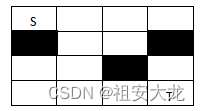小明某天不小心进入了一个迷宫（如上图所示）。请帮他判断能否出走出迷宫，如果可能，则输出一共有多少种不同的走法（对于某种特定的走法，必须保证不能多次走到同一个位置）。如果不能走到出口，则输出impossible。每次走只能是上、下、左、右4个方向之一。

输入格式:

测试数据有多组，处理到文件尾。每组测试数据首先输入2个整数n，m（0<n，m≤100），代表迷宫的高和宽，然后n行，每行m个字符，各字符的含义如下：
'S’代表小明现在所在的位置；‘T’代表迷宫的出口；’#‘代表墙，不能走；’.'代表路，可以走。

输出格式:

对于每组测试，输出一共有多少种不同的走法，若不能走出则输出impossible。

输入样例:
4 4
S…
#…#
…#.
…T
4 4
S…
#…#
…#.
…#T
输出样例:
4
impossible

代码长度限制 16 KB 时间限制 400 ms 内存限制 64 MB

解题代码

#include<bits/stdc++.h>
using namespace std;
int pos={-1,0,1,0,0,-1,0,1};	//可以走的位置
int ans;
char mg;
void findWay(int x,int y)
{
if(mg[x][y]=='T'){
ans++;
return;
}
queue<int> point;
for(int i=0;i<4;i++){
if(mg[x+pos[i]][y+pos[i]]=='.'||mg[x+pos[i]][y+pos[i]]=='T'){
point.push(x+pos[i]);
point.push(y+pos[i]);
}
}
int nx,ny;
while(!point.empty()){
nx=point.front();
point.pop();
ny=point.front();
point.pop();
mg[x][y]='#';
findWay(nx,ny);
mg[x][y]='.';	//注意回溯
}
}

int main()
{
int n,m;
int bx,by;
while(cin>>n>>m)
{
ans=0;
for(int i=0;i<=n+1;i++){
for(int j=0;j<=m+1;j++){
mg[i][j]='#';
}
}
for(int i=1;i<=n;i++){
for(int j=1;j<=m;j++){
cin>>mg[i][j];
if(mg[i][j]=='S'){
bx=i;
by=j;
}
}
}
findWay(bx,by);
if(ans==0){
cout<<"impossible"<<endl;
}else{
cout<<ans<<endl;
}
}
}


解题思路

这里用了队列去写，把当前位置周围的四个方向，能够通过的存入队列中，然后再循环出队去递归每一种可走的路径，这里要注意回溯，感觉用队列写比之前的写的要简单很多，代码冗余量比较少。最终只要能到终点就ans++，最后判断ans是不是等于0，等于0就输出impossible就可以了，否则输出ans

展开全文• 数据结构的课程设计，迷宫问题，用C++实现的，附带报告
• 数据结构基础代码，内含树，图基本操作，链表，堆栈基本操作
• 本文实例为大家分享了C++实现迷宫的具体代码，供大家参考，具体内容如下 一、 实验目的： （1） 熟练掌握链栈的基本操作及应用。 （2） 利用链表作为栈的存储结构，设计实现一个求解迷宫的非递归程序。 二、实验...
• 数据结构实验报告 迷宫求解问题实验 上机环境 DevC++ 二程序设计相关信息 1实验题目迷宫求解问题 问题描述 实验题3.5 改进3.1.4节中的求解迷宫问题程序要求输出如图3.14所示的迷宫的所有路径并求最短路径长度及最短...
• 数据结构课程设计 迷宫求解C++语言描述 这是一个数据结构的课程设计，我选择了迷宫求解 这个题目，使用C++语言才描述，经测试无误，测试环境：Windows 7 x64 旗舰版，Visual Studio 2010 旗舰版
• 摘要 本文详细介绍了迷宫问题的设计与实现 该程序具有迷宫的设计生成 逃离 迷宫的路线的寻找 打印逃离路线及标拄了逃离路线的迷宫等功能 在课程设计 中程序设计语言采用 Visual C++ 程序运行平台为 Windows 98/2000...
• C++语言写的数据机构迷宫问题数据结构课的作业。
• BFS算法迷宫 #include <iostream> #include <string> //#include <queue> #include "QueueBottom.h" #include <fstream> using namespace std; #include <iomanip> #include <...
• 迷宫问题的一个实例如下图所示。编程完成如下任务。 要求： ① 可任意指定迷宫的大小，随机产生可通和障碍方格； ② 可任意指定起始位置和目标位置； ③ 具有编辑迷宫功能，可修改当前迷宫的可通和障碍方格； ④ 计...
• 迷宫问题 C++语言 数据结构课程设计 class Point //迷宫中点位置的存储结构 { public: int x; //x代表当前位置的行坐标 int y; //y代表当前位置的列坐标 int dir; //0:无效,1:下,2:右,3:上,4:左 }; class ...
• 本资源提供数据结构（清华大学出版社）——迷宫（递归）的完整代码
• 在学习数据结构中自己实现的迷宫游戏。这个代码中有迷宫生成（迷宫比较不错），然后对生成的迷宫用递归算法寻找路径。在迷宫设计以及递归学习是个不错的选择。
• 以一个m×n的长方阵表示迷宫，0和1分别表示迷宫中的通路和障碍。设计一个程序，对信任意设定的迷宫，求出一条从入口到出口的通路，或得出没有通路的结论。
• 可以手动或者自动生成一个迷宫。自动生成的迷宫必有且只有一条通路，然后可以动态地逐步寻找到通路。
• 此项目只是为了在毕业之前复习一下曾经学过的数据结构/算法，初衷是打算仅仅使用Python实现，但写了简单的排序/查找算法之后，觉得对于C/C++我还是应该学习一下，于是就附带了一些之前在BNU上的练习代码。......

# 数据结构迷宫问题c++c++ 订阅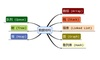数据结构 订阅